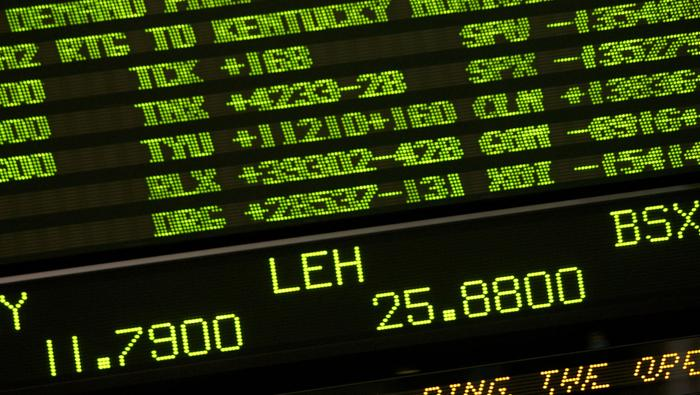News & Analysis at your fingertips.

We use a range of cookies to give you the best possible browsing experience. By continuing to use this website, you agree to our use of cookies.

0

#### Economic Calendar Events

0

Live Webinar Events
Economic Calendar Events

H

High

M

Medium

L

Low
EUR/USD
Bullish
Low
High
Long Short

Note: Low and High figures are for the trading day.

Data provided by
Oil - US Crude
Bearish
Low
High
Long Short

Note: Low and High figures are for the trading day.

Data provided by
Wall Street
Bearish
Low
High
Long Short

Note: Low and High figures are for the trading day.

Data provided by
Gold
Mixed
Low
High
Long Short

Note: Low and High figures are for the trading day.

Data provided by
GBP/USD
Bearish
Low
High
Long Short

Note: Low and High figures are for the trading day.

Data provided by
USD/JPY
Bullish
Low
High
Long Short

Note: Low and High figures are for the trading day.

Data provided by# Fibonacci Application in Financial Markets

The word “Fibonacci” often appears in trading and it is likely that you have already seen or heard of it - for good reason. Fibonacci numbers are numbers that are arranged according to a specific formula to create a unique numerical sequence. Within the sequence lies the Golden Ratio of 1.618, which appears frequently throughout nature and is also used extensively in technical analysis, along with other Fibonacci numbers and ratios, to help identify areas of support and resistance.Recommended by Richard Snow
Forex for Beginners

## Fibonacci Foundations, Applications in the World Around US

Fibonacci numbers get their name from the Italian mathematician who discovered them, ‘ Leonardo Fibonacci’ in the thirteenth century. While his book “Liber Abaci” introduced the number sequence to the Western world, traces of it can be found as early as 200 BC in Indian mathematics.

The number sequence starts with 1 and each number in the sequence can be arrived at by adding the two previous numbers in the sequence.

Fibonacci sequence: 0,1,1,2,3,5,8,13,21,34,55,89,144,233,377,610,987,1597,2584,4181,765,1094,17711

Fibonacci in nature:

Sunflower seeds have a very distinct spiral pattern to their make-up. Later on in the math section, we discuss the spiral feature of the Fibonacci sequence. Also, sunflowers tend to have 34 spirals in total, a Fibonacci number.Daisies are typically found to have 34, 55 or 89 petals – all Fibonacci numbers.Extreme weather patterns have a tendency to form spirals, therefore, their very structure comprises of Fibonacci.Other examples where Fibonacci numbers can be observed, include: music, art (Mona Lisa), architecture and biology. Right now, you can look down at your hands to notice that you probably have eight fingers, five digits on each hand, three bones in each finger, two bones in each one thumb and one thumb on each hand (8, 5, 3, 2, 1, 1).Recommended by Richard Snow

## Fibonacci: The Math

The diagram below shows a visual representation of the Fibonacci spiral, often seen in nature, when applying it to area calculations. For example, the spiral starts from within a 1x1 square (the first Fibonacci number is 1), then moves to another 1x1 square (the second Fibonacci number is also 1), before moving on to a 2x2 square (the third Fibonacci number) and so on. The spiral is seen when connecting each additional square by drawing a curved line through the diagonal of each new square.Fibonacci numbers are fairly significant on their own, however when analyzing the ratios that exist among the infinite number sequence, the real value of Fibonacci becomes clear.

The Golden Ratio:

Take any number in the sequence and divide it by the number before it and the answer will be 1.618, or close to this number for the numbers from 1 to 55 in the sequence. This is often referred to by the Greek letter ϕ “Phi”.

Examples:

a/b = ϕ

17711/10946 = 1.61803

6765/4181 = 1.61803

21/13 = 1.6154 (less approximate for numbers 55 and lower)

## Fibonacci Application in Financial Markets

While the application of Fibonacci in nature keeps many graduate level mathematics students busy, traders have more pressing concerns: Applying the study to financial markets. In its most common form, Fibonacci makes use of mathematically relevant ratios to project possible areas/zones of support and resistance.Recommended by Richard Snow

Refer to the USD/SGD daily chart below as an example where the Fibonacci retracement drawing tool has been applied to a significant move (bottom to top). To reduce clutter on the chart you will notice only the 0, 38.2, 50, 61.8 and 100 percent markers are shown.From the peak, the drawing tool shows price action struggling to make a clear directional break below the 38.2% level – the reciprocal of the 61.8% ( 1 - 0.618 ). Price hovered around until a decisive breakdown toward the 61.8% level which acted as support.

This example shows how the 38.2 and 50 percent levels are less significant than the 61.8% level - which acted as a major level of support. While Fibonacci levels are not perfect, meaning price action may move through the defined levels, it is still a very useful indicator revealing where a particular market may run into support and resistance or consolidate before breaking into a fresh trend.

DailyFX provides forex news and technical analysis on the trends that influence the global currency markets.